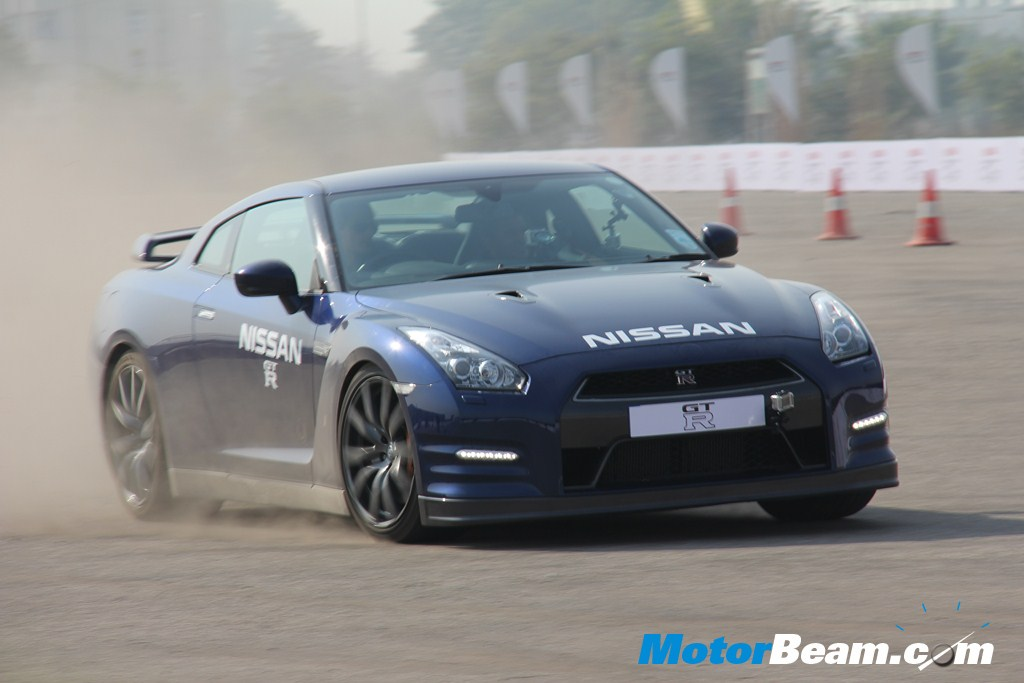Nissan GT-R Arrives In India - Click above for picture gallery

Nissan has got the 2011 GT-R to India for the 2012 Auto Expo. This value for money supercar is filled with technology and is can out run cars which are more than double it’s price. Powered by a 3.8-liter, VR38DETT twin-turbo V6 motor, the 2011 GT-R produces The V-spec variant has a high-gear boost option which increases the torque to 632 Nm. With a drag co-efficient of just 0.26, the GT-R is as slippery as you can get. One of the smart features of the Nissan GT-R is the launch control, which propels the car to 100 kmph in just 3.2 seconds. Top speed is 315 kmph ad the GT-R has a host of electronic systems to ensure high levels of grip at speed. In this post, we will let the pictures do the talking, so if you want to read more about the Nissan GT-R, you should head here.

[singlepic id=8853 w=540 h=375 float=center]
[singlepic id=8846 w=540 h=375 float=center]
[singlepic id=8873 w=540 h=375 float=center]
[singlepic id=8847 w=540 h=375 float=center]
[singlepic id=8856 w=540 h=375 float=center]
[singlepic id=8852 w=540 h=375 float=center]
[singlepic id=8843 w=540 h=375 float=center]
[singlepic id=8877 w=540 h=375 float=center]
[singlepic id=8845 w=540 h=375 float=center]
[singlepic id=8863 w=540 h=375 float=center]
[singlepic id=8867 w=540 h=375 float=center]
[singlepic id=8854 w=540 h=375 float=center]
[singlepic id=8859 w=540 h=375 float=center]
[singlepic id=8881 w=540 h=375 float=center]
[singlepic id=8860 w=540 h=375 float=center]
[singlepic id=8876 w=540 h=375 float=center]